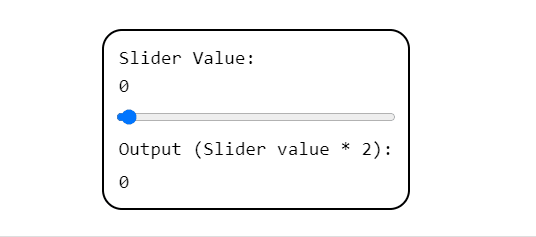# <output>

The `<output>` element displays the result of a calculation or user action. It is commonly used inside of a `<form>` element to dynamically show the result of user input. However, the `<output>` element can be used on its own.

## Syntax

```The result of 5 + 5 is <output name="operation-result">10</output>
```

The `<output>` element requires opening and closing tags that wrap around the result.

### Attributes

The `<output>` element takes the following attributes:

• `for` indicates the id of one or multiple elements (separated by a space) whose values are factored in the calculation.
• `form` indicates one or more forms the `<output>` element belongs to.
• `name` indicates the name of the element.

## Example

The example form below features an `<output>` element displaying the slider value multiplied by two:

```<html>  <head> </head>  <body>    <form      oninput="result.value=parseInt(sliderInput.value)*2; document.querySelector('span').innerText=sliderInput.value"      id="calculation-form"    >      <label for="slideInput" id="sliderValue">        Slider Value: <span> </span>      </label>      <input name="sliderInput" type="range" value="0" />      <label for="result"> Output (Slider value * 2): </label>      <output name="result" for="sliderInput" form="calculation-form"> </output>    </form>  </body></html>
```